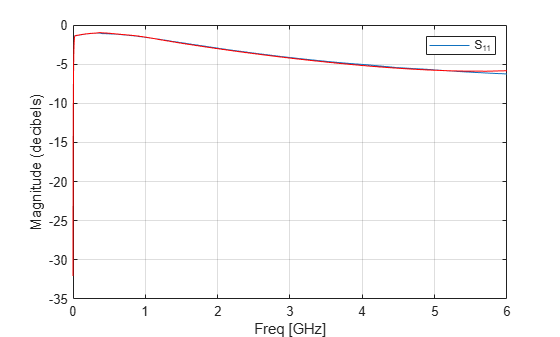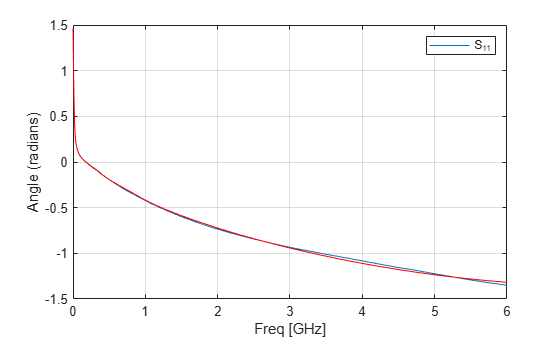# rationalfit

Approximate data using stable rational function object

## Syntax

``fit = rationalfit(freq,data)``
``fit = rationalfit(freq,data,tol)``
``fit = rationalfit(___,Name,Value)``
``[fit,errdb] = rationalfit(...)``
``fit = rationalfit(s_obj,i,j)``

## Description

example

````fit = rationalfit(freq,data)` fits a rational function object of the form$\begin{array}{cc}F\left(s\right)=\sum _{k=1}^{n}\frac{{C}_{k}}{s-{A}_{k}}+D,& s=j*2\pi f\end{array}$to the complex vector `data` over the frequency values in the positive vector `freq`. The function returns a handle to the rational function object, `h`, with properties `A`, `C`, `D`, and `Delay`.```
````fit = rationalfit(freq,data,tol)` fits a rational function object to complex data and constrains the error of the fit according to the optional input argument `tol`.```
````fit = rationalfit(___,Name,Value)` fits a rational function object of the form $F\left(s\right)=\left(\sum _{k=1}^{n}\frac{{C}_{k}}{s-{A}_{k}}+D\right){e}^{-s.Delay},\text{ }\text{\hspace{0.17em}}\text{\hspace{0.17em}}s=j*2\pi f$with additional options specified by one or more `Name,Value` pair arguments. These arguments offer finer control over the performance and accuracy of the fitting algorithm.```
````[fit,errdb] = rationalfit(...)` fits a rational function object to complex data and also returns `ERRDB`, which is the achieved error.```

example

````fit = rationalfit(s_obj,i,j)` fits `Sij` using ```FREQ = s_obj.Frequencies``` and `DATA = rfparam(s_obj,i,j)` for s-parameter object, `s_obj`.```

## Examples

collapse all

Fit a rational function object to S-parameter data, and compare the results by plotting the object against the data.

Read the S-parameter data into an RF data object.

```orig_data = read(rfdata.data,'passive.s2p'); freq = orig_data.Freq; data = orig_data.S_Parameters(1,1,:);```

Fit a rational function to the data using `rationalfit`.

`fit_data = rationalfit(freq,data)`
```fit_data = rfmodel.rational with properties: A: [19x1 double] C: [19x1 double] D: 0 Delay: 0 Name: 'Rational Function' ```

Compute the frequency response of the rational function using `freqresp`.

`[resp,freq] = freqresp(fit_data,freq);`

Plot the magnitude of the original data against the rational function approximation. ${S}_{11}$ data appears in blue, and the rational function appears in red. Scaling the frequency values by `1e9` converts them to units of GHz.

```figure title('Rational fitting of S11 magnitude') plot(orig_data,'S11','dB') hold on plot(freq/1e9,20*log10(abs(resp)),'r');```Plot the angle of the original data against the rational function approximation.

```figure title('Rational fitting of S11 angle') plot(orig_data,'S11','Angle (radians)') hold on plot(freq/1e9,unwrap(angle(resp)),'r')````rationalfit(freq,data)` also handles input 3D array of data (n x n x p), an input frequency array (p x 1), and returns a matrix (n x n) of rationalfit objects. Index into the matrix of rationalfit objects to access corresponding rationalfit information.

Use `rationalfit` on multiple datasets defined in a matrix.

```orig_data = sparameters('defaultbandpass.s2p'); data = orig_data.Parameters; freq = orig_data.Frequencies; fit_data = rationalfit(freq, data)```
```fit_data=2×2 object 2x2 rfmodel.rational array with properties: A C D Delay Name ```

To access `rationalfit` data, use indexing on the rationalfit array. For example, to access the rational fit for the 1st element of the matrix, use:

`S = fit_data(1, 1)`
```S = rfmodel.rational with properties: A: [12x1 double] C: [12x1 double] D: 0 Delay: 0 Name: 'Rational Function' ```

Use rational fit to fit an S-parameter object from the file 'passive.s2p'.

```S = sparameters('passive.s2p'); fit = rationalfit(S,1,1,'TendsToZero',false)```
```fit = rfmodel.rational with properties: A: [5x1 double] C: [5x1 double] D: -0.4843 Delay: 0 Name: 'Rational Function' ```

## Input Arguments

collapse all

Frequencies over which the function fits a rational object, specified as a vector of length M.

Data to fit, specified as an N-by-N-by-M array of complex numbers. The function fits N2 rational functions to the data along the M (frequency) dimension.

Error tolerance ε, specified as a scalar in units of dB. The error-fitting equation is

`${10}^{\epsilon /20}\ge \frac{\sqrt{\sum _{k=0}^{n}{|{W}_{k}{F}_{0}\left\{{f}_{k}\right\}-F\left(s\right)|}^{2}}}{\sqrt{\text{ }\sum _{k=0}^{n}{|{W}_{k}{F}_{0}\left\{{f}_{k}\right\}|}^{2}}}$`

where

• ε is the specified value of `tol`.

• F0 is the value of the original data (`data`) at the specified frequency fk (`freq`).

• F is the value of the rational function at s = j2πf.

• W is the weighting of the data.

`rationalfit` computes the relative error as a vector containing the dependent values of the fit data. If the object does not fit the original data within the specified tolerance, a warning message appears.

S-parameters, specified as an RF Toolbox™ network parameter object. To create this type of object, use the `sparameters` function.

Row index of data to plot, specified as a positive integer.

Column index of data to plot, specified as a positive integer.

### Name-Value Pair Arguments

Specify optional comma-separated pairs of `Name,Value` arguments. `Name` is the argument name and `Value` is the corresponding value. `Name` must appear inside quotes. You can specify several name and value pair arguments in any order as `Name1,Value1,...,NameN,ValueN`.

Example: `'DelayFactor',0.2`

Scaling factor that controls the amount of delay to fit to the data, specified as the comma-separated pair consisting of `'DelayFactor'` and a scalar between 0 and 1 inclusive. The `Delay` parameter, τ, of the rational function object is equal to the specified value of `'DelayFactor'` times an estimate of the group delay of the data. If the original data has delay, increasing this value might allow `rationalfit` to fit the data with a lower-order object.

Maximum number of `rationalfit` iterations, specified as a vector of positive integers. Provide a two-element vector to specify minimum and maximum `[M1 M2]`. Increasing the limit extends the time that the algorithm takes to produce a fit, but it might produce more accurate results.

Number of poles Ak of the rational function, specified as the comma-separated pair consisting of `'NPoles'` and an integer n or range of possible values of n.

To help `rationalfit` produce an accurate fit, choose a maximum value of `npoles` greater than or equal to twice the number of peaks on a plot of the data in the frequency domain.

After completing a rational fit, the function removes coefficient sets whose residues (Ck) are zero. Thus, when you specify a range for `npoles`, the number of poles of the fit may be less than `npoles(1)`.

Asymptotic behavior of the rational function as frequency approaches infinity, specified as the comma-separated pair consisting of `'TendsToZero'` and a logical value. When this argument is `true`, the resulting rational function variable D is zero, and the function tends to zero. A value of `false` allows a nonzero value for `D`.

Error tolerance ε, specified as the comma-separated pair consisting of `'Tolerance'` and a scalar in units of dB. The error-fitting equation is

`${10}^{\epsilon /20}\ge \frac{\sqrt{\sum _{k=0}^{n}{|{W}_{k}{F}_{0}\left\{{f}_{k}\right\}-F\left(s\right)|}^{2}}}{\sqrt{\text{ }\sum _{k=0}^{n}{|{W}_{k}{F}_{0}\left\{{f}_{k}\right\}|}^{2}}}$`

where

• ε is the specified tolerance.

• F0 is the value of the original data (`data`) at the specified frequency fk (`freq`).

• F is the value of the rational function at s = j2πf.

• W is the weighting of the data.

If the object does not fit the original data within the specified tolerance, the function throws a warning.

Logical value that toggles display of the graphical wait bar during fitting, specified as the comma-separated pair consisting of `'WaitBar'` and either `true` or `false`. The `true` setting shows the graphical wait bar, and the `false` setting hides it. If you expect `rationalfit` to take a long time, and you want to monitor its progress, set `'WaitBar'` to `true`.

Weighting of the data at each frequency, specified as the comma-separated pair consisting of `'Weight'` and a vector of positive numbers or an array same as that of the data. Each entry in `weight` corresponds to a frequency in `freq`, so the length of `weight` must be equal to the length of `freq`. Increasing the weight at a particular frequency improves the object fitting at that frequency. Specifying a weight of `0` at a particular frequency causes `rationalfit` to ignore the corresponding data point.

## Output Arguments

collapse all

One or more rational function objects, returned as an N-by-N `rfmodel.rational` object. The number of dimensions in `data` determines the dimensionality of `h`.

Relative error achieved, returned as a `double`, in dB.

## Tips

To see how well the object fits the original data, use the `freqresp` function to compute the frequency response of the object. Then, plot the original data and the frequency response of the rational function object. For more information, see the `freqresp` reference page or the above examples.

 Gustavsen.B and A.Semlyen, “Rational approximation of frequency domain responses by vector fitting,” IEEE Trans. Power Delivery, Vol. 14, No. 3, pp. 1052–1061, July 1999.

 Zeng.R and J. Sinsky, “Modified Rational Function Modeling Technique for High Speed Circuits,” IEEE MTT-S Int. Microwave Symp. Dig., San Francisco, CA, June 11–16, 2006.